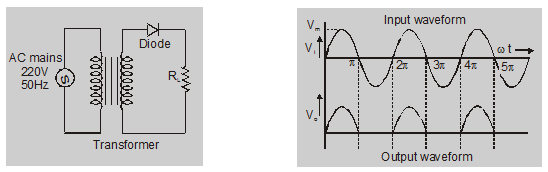Deepak Scored 45->99%ile with Bounce Back Crack Course. You can do it too!

# Half wave rectifier circuit diagram - Definition, Explanation - eSaral

A device that converts alternating current into Direct current is called a rectifier. If you want to learn about the half-wave rectifier and half-wave rectifier circuit diagram then you are at the right place.

## Application of diode as a rectifier:

An electronic device that converts alternating current into Direct current is called a rectifier.

### Half wave rectifier:

A rectifier, which rectifies only one half of each ac supply cycle is called a half-wave rectifier.During the first half of the input cycle, the junction diode gets forward bias. The conventional current will flow. The upper end of $R_{L}$ will be positive potential with respect to the lower end during the second half cycle junction diode will get reverse biased and hence no output will be obtained across $R_{L}$.

Input voltage

$\mathrm{V}_{\mathrm{i}}=\mathrm{V}_{\mathrm{m}} \sin \omega \mathrm{t}$

$\mathrm{i}=\mathrm{I}_{\mathrm{m}} \sin \omega \mathrm{t}$

for $0 \leq \omega t \leq \pi$

$\mathrm{i}=0$

for $\pi<\omega t<2 \pi$

$I_{m}=\frac{V_{m}}{R_{f}+R_{L}}$

here $\mathrm{R}_{\mathrm{f}}=$ forward resistance of diode

$R_{L}$ = load resistance

a. dc output current :

$\mathrm{I}_{\mathrm{dc}}=\frac{1}{2 \pi} \int_{0}^{2 \pi} \mathrm{idt}$

$=\frac{1}{2 \pi}\left[\int_{0}^{\pi} \mathrm{I}_{\mathrm{m}} \sin t \mathrm{dt}+\int_{\pi}^{2 \pi} 0 \mathrm{dt}\right]$

$\mathrm{I}_{\mathrm{dc}}=\frac{\mathrm{I}_{\mathrm{m}}}{\pi}=0.318 \mathrm{I}_{\mathrm{m}}$

b. dc output voltage:

$V_{d c}=I_{d c} \times R_{L}$

$=\frac{I_{m}}{\pi} \times R_{L}$

$=\frac{\mathrm{V}_{\mathrm{m}}}{\pi\left[1+\left(\mathrm{R}_{\mathrm{f}} / \mathrm{R}_{\mathrm{L}}\right)\right]}$

$V_{d c}=\frac{V_{m}}{\pi}=0.318 \mathrm{~V}_{\mathrm{m}}$

c. (Root mean square) RMS current:

$I_{r m s}=\left[\frac{1}{2 \pi} \int_{0}^{2 \pi} i^{2} d(t)\right]^{1 / 2}$

$=\frac{I_{m}}{2}$

same

$V_{r m s}=\frac{V_{m}}{2}$

So, that's all from this blog. I hope you enjoyed this explanation of the half-wave rectifier and half-wave rectifier circuit diagram. If you liked this article then please share it with your friends.